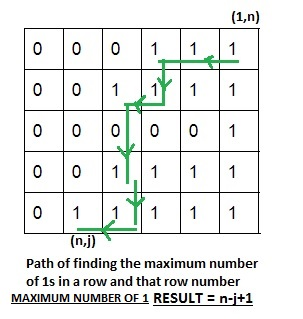# Find row number of a binary matrix having maximum number of 1s

• Difficulty Level : Medium
• Last Updated : 18 Jul, 2022

Given a binary matrix (containing only 0 and 1) of order n×n. All rows are sorted already, We need to find the row number with the maximum number of 1s. Also, find the number of 1 in that row.

Note: in case of a tie, print the smaller row number.

Examples :

```Input : mat = {0, 0, 1,
0, 1, 1,
0, 0, 0}
Output : Row number = 2, MaxCount = 2

Input : mat = {1, 1, 1,
1, 1, 1,
0, 0, 0}
Output : Row number = 1, MaxCount = 3```

Basic Approach: Traverse whole of matrix and for each row find the number of 1 and among all that keep updating the row number with the maximum number of 1. This approach will result in O(n^2) time complexity.

Better Approach: We can perform better if we try to apply the binary search for finding the position of the first 1 in each row and as per that we can find the number of 1 from each row as each row is in sorted order. This will result in O(nlogn) time complexity.

Efficient Approach: Start with the top right corner with index (1, n) and try to go left until you reach the last 1 in that row (jth column), now if we traverse left to that row, we will find 0, so switch to the row just below, with the same column. Now your position will be (2, j) again in the 2nd row if the jth element is 1 try to go left until you find the last 1 otherwise in the 2nd row if jth element is 0 goes to the next row. So Finally say if you are at any ith row and jth column which is the index of last 1 from right in that row, increment i. So now if we have Aij = 0 again increment i otherwise keep decreasing j until you find the last 1 in that particular row.
Sample Illustration :Algorithm :

```for (int i=0, j=n-1; i<n;i++)
{
// find left most position of 1 in a row
// find 1st zero in a row
while (arr[i][j]==1)
{
row = i;
j--;
}
}
cout << "Row number =" << row+1;
cout << "MaxCount =" << n-j;```

Implementation:

## C++

 `// CPP program to find row with maximum 1``// in row sorted binary matrix``#include``#define N 4``using` `namespace` `std;` `// function for finding row with maximum 1``void` `findMax (``int` `arr[][N])``{``    ``int` `row = 0, i, j;``    ``for` `(i=0, j=N-1; i= 0)``        ``{``            ``row = i;``            ``j--;``        ``}``    ``}``    ``cout << ``"Row number = "` `<< row+1;``    ``cout << ``", MaxCount = "` `<< N-1-j;``}` `// driver program``int` `main()``{``    ``int` `arr[N][N] = {0, 0, 0, 1,``                     ``0, 0, 0, 1,``                     ``0, 0, 0, 0,``                     ``0, 1, 1, 1};``    ``findMax(arr);``    ``return` `0;``}`

## Java

 `// Java program to find row with maximum 1``// in row sorted binary matrix``class` `GFG {``    ` `    ``static` `final` `int` `N = ``4``;` `    ``// function for finding row with maximum 1``    ``static` `void` `findMax(``int` `arr[][]) {``        ` `        ``int` `row = ``0``, i, j;` `        ``for` `(i = ``0``, j = N - ``1``; i < N; i++) {``            ` `            ``// find left most position of 1 in``            ``// a row find 1st zero in a row``            ``while` `(j >= ``0` `&& arr[i][j] == ``1``) {``                ` `                ``row = i;``                ``j--;``            ``}``        ``}``        ` `        ``System.out.print(``"Row number = "``                                ``+ (row + ``1``));``        ``System.out.print(``", MaxCount = "``                               ``+ (N - ``1` `- j));``    ``}``    ` `    ``// Driver code``    ``public` `static` `void` `main(String[] args) {``        ``int` `arr[][] = {{``0``, ``0``, ``0``, ``1``},``                       ``{``0``, ``0``, ``0``, ``1``},``                       ``{``0``, ``0``, ``0``, ``0``},``                       ``{``0``, ``1``, ``1``, ``1``}};``        ``findMax(arr);``    ``}``}` `// This code is contributed by Anant Agarwal.`

## Python3

 `# python program to find row with``# maximum 1 in row sorted binary``# matrix` `N ``=` `4` `# function for finding row with``# maximum 1``def` `findMax (arr):``    ``row ``=` `0``    ``j ``=` `N ``-` `1``    ``for` `i ``in` `range``(``0``, N):``        ``# find left most position``        ``# of 1 in a row find 1st``        ``# zero in a row``        ``while` `(arr[i][j] ``=``=` `1``                     ``and` `j >``=` `0``):``            ``row ``=` `i``            ``j ``-``=` `1``        ` `    ``print``(``"Row number = "` `, row ``+` `1``,``         ``", MaxCount = "``, N ``-` `1` `-` `j)` `# driver program``arr ``=` `[ [``0``, ``0``, ``0``, ``1``],``        ``[``0``, ``0``, ``0``, ``1``],``        ``[``0``, ``0``, ``0``, ``0``],``        ``[``0``, ``1``, ``1``, ``1``] ]``        ` `findMax(arr)` `# This code is contributed by Sam007`

## C#

 `// C# program to find row with maximum``// 1 in row sorted binary matrix``using` `System;` `class` `GFG {``    ` `    ``static` `int` `N = 4;` `    ``// function for finding row with maximum 1``    ``static` `void` `findMax(``int` `[,]arr)``    ``{``        ``int` `row = 0, i, j;` `        ``for` `(i = 0, j = N - 1; i < N; i++) {``            ` `            ``// find left most position of 1 in``            ``// a row find 1st zero in a row``            ``while` `(arr[i,j] == 1 && j >= 0)``            ``{``                ``row = i;``                ``j--;``            ``}``        ``}``        ` `        ``Console.Write(``"Row number = "` `+ (row + 1));``        ``Console.Write(``", MaxCount = "` `+ (N - 1 - j));``    ``}``    ` `    ``// Driver code``    ``public` `static` `void` `Main()``    ``{``        ``int` `[,]arr = {{0, 0, 0, 1},``                      ``{0, 0, 0, 1},``                      ``{0, 0, 0, 0},``                      ``{0, 1, 1, 1}};``        ``findMax(arr);``    ``}``}` `// This code is contributed by nitin mittal`

## PHP

 `= 0)``        ``{``            ``\$row` `= ``\$i``;``            ``\$j``--;``        ``}``    ``}``    ``echo` `"Row number = "` `, ``\$row` `+ 1;``    ``echo` `", MaxCount = "` `, ``\$N` `- 1 - ``\$j``;``}` `    ``// Driver Code``    ``\$arr` `= ``array``(``array``(0, 0, 0, 1),``                 ``array``(0, 0, 0, 1),``                 ``array``(0, 0, 0, 0),``                 ``array``(0, 1, 1, 1));``    ``findMax(``\$arr``);` `// This code is contributed by vt_m.``?>`

## Javascript

 ``

Output

`Row number = 4, MaxCount = 3`

Time Complexity: O(N2)
Auxiliary Space: O(1)